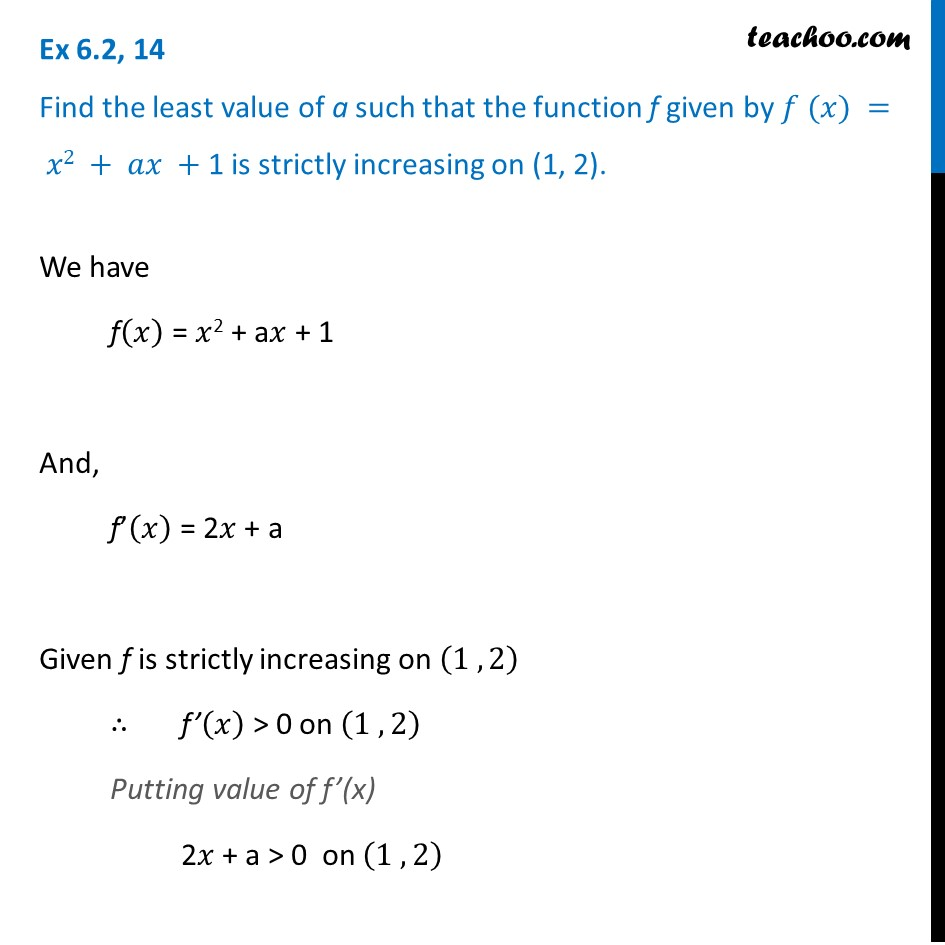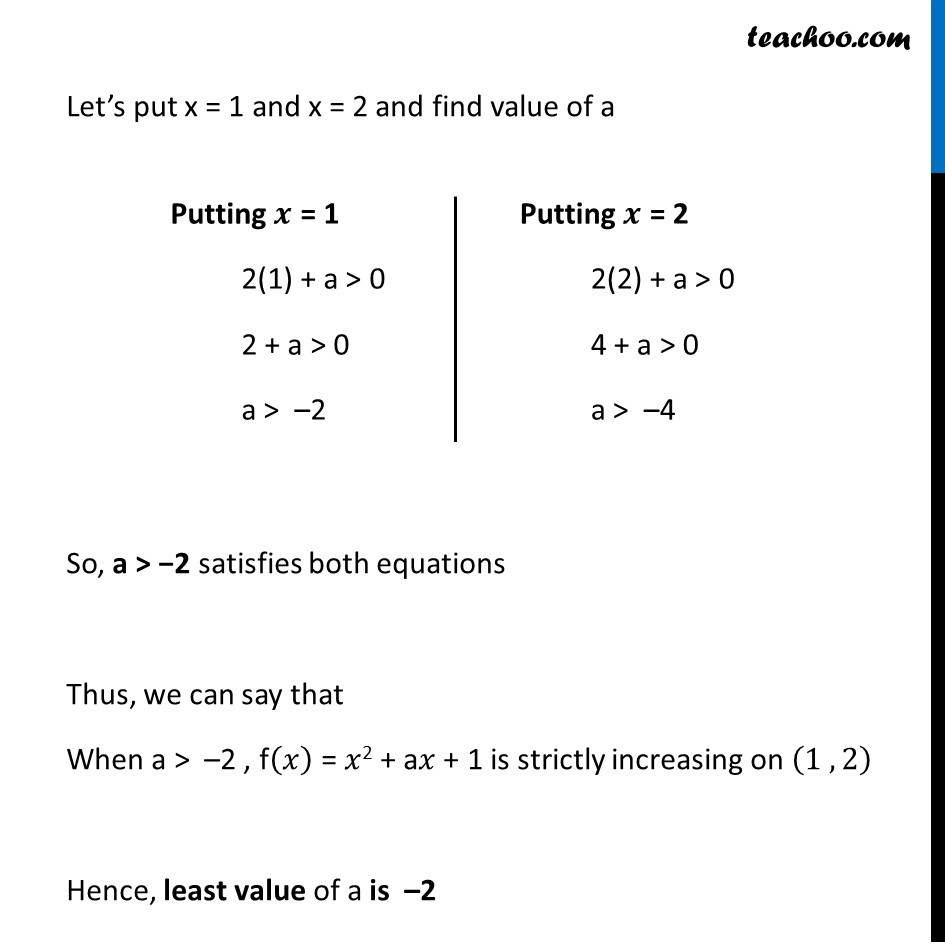Ex 6.2

Chapter 6 Class 12 Application of Derivatives
Serial order wiseLearn in your speed, with individual attention - Teachoo Maths 1-on-1 Class

### Transcript

Ex 6.2, 14 Find the least value of a such that the function f given by 𝑓 (𝑥) = 𝑥2 + 𝑎𝑥 + 1 is strictly increasing on (1, 2).We have f(𝑥) = 𝑥2 + a𝑥 + 1 And, f’(𝑥) = 2𝑥 + a Given f is strictly increasing on (1 ,2) ∴ f’(𝑥) > 0 on (1 ,2) Putting value of f’(x) 2𝑥 + a > 0 on (1 ,2) Let’s put x = 1 and x = 2 and find value of a So, a > −2 satisfies both equations Thus, we can say that When a > –2 , f(𝑥) = 𝑥2 + a𝑥 + 1 is strictly increasing on (1 , 2) Hence, least value of a is –2 Putting 𝒙 = 1 2(1) + a > 0 2 + a > 0 a > –2 Putting 𝒙 = 2 2(2) + a > 0 4 + a > 0 a > –4